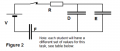# Capacitors in series and parallel help.

#### Terry Langman

Joined Jan 16, 2018
3
Hi, ive been given three circuits to find the voltage, charge and energy across three capacitors. My first circuit was three parallel and second circuit was three series capacitors. These two i have not had any problem solving but im struggling with my third circuit which has both series and parallel.This is the circuit im struggling with.
Voltage=85v
D=0.80mF
E=1.3mF
F=6mF

Using this information i must find voltage, charge and energy.

#### MrChips

Joined Oct 2, 2009
29,236
Calculate the equivalent capacitance of all three capacitances put together.
If you studied the first two circuits you would know how to do the third circuit.

#### Terry Langman

Joined Jan 16, 2018
3
i dont know how to do this as the first two circuits i studied was either parallel or series. The parallel circuit i added the capacitors up then multiplied the voltage to get the charge. But with the series it was a different way so how do i do these 2 combined in one circuit .

#### smooth_jamie

Joined Jan 4, 2017
107
Calculate capacitors D and E in parallel and then that equivalent cap (call it DE) will be in series with F. Then just calculate DE and F as two caps in series.

#### WBahn

Joined Mar 31, 2012
29,166
i dont know how to do this as the first two circuits i studied was either parallel or series. The parallel circuit i added the capacitors up then multiplied the voltage to get the charge. But with the series it was a different way so how do i do these 2 combined in one circuit .
Can you take the two caps that are in parallel (forget everything else) and replace them by a single capacitor that is equivalent to them. Redraw the circuit with this modification. Does the circuit now look like something familiar?

#### Terry Langman

Joined Jan 16, 2018
3
I can do this yeah, but its asking me to find the charge and energy across all capacitors so if i put the parallel into a single capacitor i can only find the values for two capacitors not three.

#### WBahn

Joined Mar 31, 2012
29,166
I can do this yeah, but its asking me to find the charge and energy across all capacitors so if i put the parallel into a single capacitor i can only find the values for two capacitors not three.
Let's say that I have a 4 uF cap and a 2 uF cap in parallel. The combined capacitance is 6 uF, right?

Now I use that combined capacitance to analyze a circuit and conclude that the voltage across the 6 uF equivalent is 12 V.

Can you figure out how much charge and energy is stored on the original 4 uF and 2 uF caps?